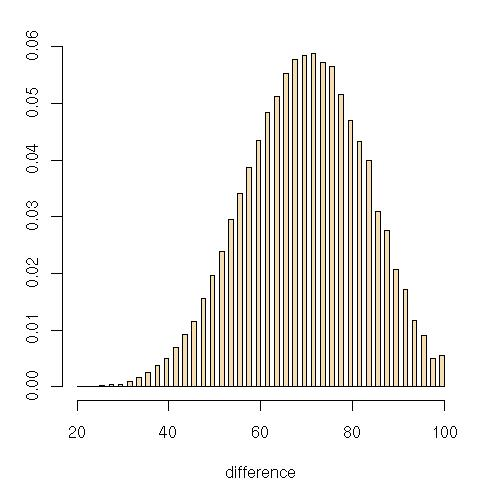Want to share your content on R-bloggers? click here if you have a blog, or here if you don't.

In the puzzle found in Le Monde of this weekend, the mathematical object behind the silly story is defined as a pseudo-Spearman rank correlation test statistic,$mathfrak{M}_n = sum_{i=1}^n |r^x_i-r^y_i|,,$

where the difference between the ranks of the paired random variables$x_i$ and$y_i$ is in absolute value instead of being squared as in the Spearman rank test statistic. I don’t know whether or not this measure of distance has been studied in the statistics literature (although I’d be surprised has it not been studied!). Here is an histogram of the distribution of the new statistics for$n=20$ under the null hypothesis that both samples are uncorrelated (i.e. that the sequence of ranks is a random permutation). Each point in the sample was obtained by

```perm=sample(1:20)
saple[t]=sum(abs(perm[1:10]-perm[11:20]))```When regressing the mean of this statistic$mathfrak{M}_n$ against the covariates$n$ and$n^2$, I obtain the uninspiring formula$mathbb{E} [mathfrak{M}_n] approx 0.1681 n^2 - 0.3769 n + 11.1921$

which does not translate into a nice polynomial in$n$!

Another interesting probabilistic/combinatorial problem issued from an earlier Le Monde puzzle: given an urn with$n$ white balls and$n$ black balls that is sampled without replacement, what is the probability that there exists a sequence of length$2k$ with the same number of white and black balls for$k=1,ldots,n$? If$k=1,n$, the answer is obviously one (1), but for some values of$k$, it is less than one. When$n$ goes to infinity, this is somehow related to the probability that a Brownian bridge crosses the axis in-between$0$ and$1$ but I have no clue whether this helps or not! Robin Ryder solved the question for the values$n=50$ and$k=24,25$ by establishing that the probability is still one.

Ps- The same math tribune in Le Monde coincidently advertises a book, Le Mythe Climatique, by Benoît Rittaud that adresses … climate change issues and the “statistical mistakes made by climatologists”. The interesting point (if any) is that Benoît Rittaud is a “mathematician not a statistician”, with a few papers in ergodic theory, but this advocated climatoskeptic nonetheless criticises the use of both statistical and simulation tools in climate modeling. (“Simulation has only been around for a few dozen years, a very short span in the history of sciences. The climate debate may be an opportunity to reassess the role of simulation in the scientific process.”)

Filed under: R, Statistics Tagged: climate change, climatosceptic, correlation, global warming, Le Monde, puzzle, R, Robin Ryder, Spearman rank test